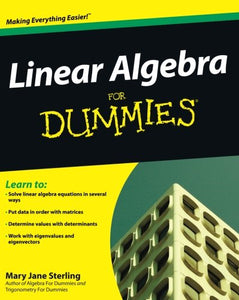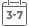# Linear Algebra For Dummies

• Publish Date: 2009-07-07
• Binding: Paperback
• Author: Mary Jane Sterling
Vendor
Sterling, Mary Jane
Regular price
\$33.11
Sale price
\$19.77
Quantity must be 1 or more

Attention: For textbook, access codes and supplements are not guaranteed with used items.Learn to:

• Solve linear algebra equations in several ways
• Put data in order with matrices
• Determine values with determinants
• Work with eigenvalues and eigenvectors

Your hands-on guide to real-world applications of linear algebra

Does linear algebra leave you feeling lost? No worries this easy-to-follow guide explains the how and the why of solving linear algebra problems in plain English. From matrices to vector spaces to linear transformations, you'll understand the key concepts and see how they relate to everything from genetics to nutrition to spotted owl extinction.

• Line up the basics discover several different approaches to organizing numbers and equations, and solve systems of equations algebraically or with matrices
• Relate vectors and linear transformations link vectors and matrices with linear combinations and seek solutions of homogeneous systems
• Evaluate determinants see how to perform the determinant function on different sizes of matrices and take advantage of Cramer's rule
• Hone your skills with vector spaces determine the properties of vector spaces and their subspaces and see linear transformation in action
• Tackle eigenvalues and eigenvectors define and solve for eigenvalues and eigenvectors and understand how they interact with specific matrices

Open the book and find:

• Theoretical and practical ways of solving linear algebra problems
• Definitions of terms throughout and in the glossary
• New ways of looking at operations
• How linear algebra ties together vectors, matrices, determinants, and linear transformations
• Ten common mathematical representations of Greek letters
• Real-world applications of matrices and determinants